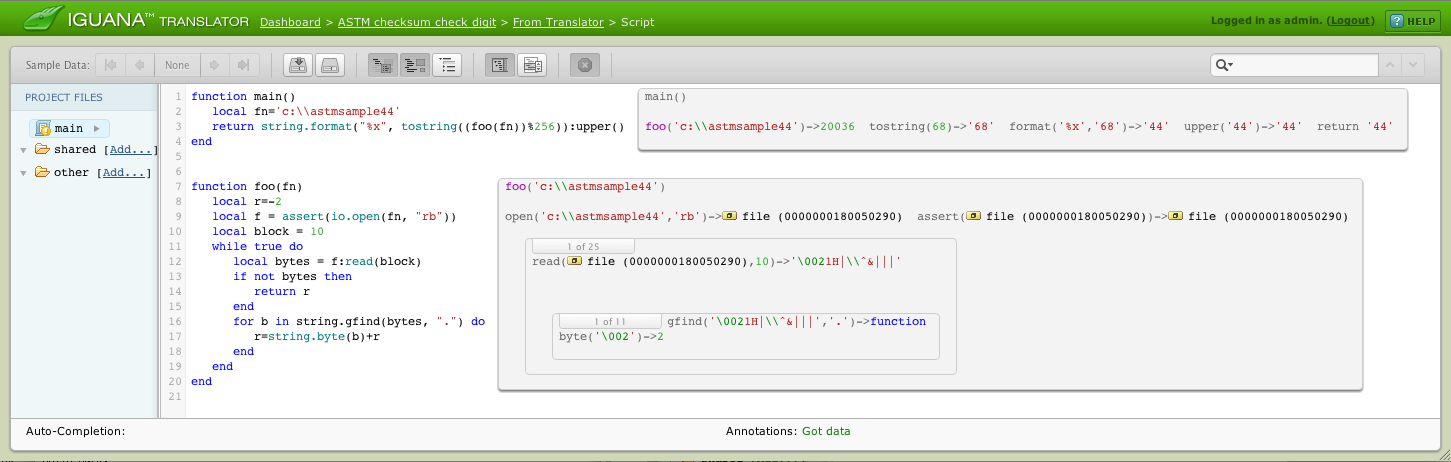# How to calculate ASTM checksum for outbound or resumbitted message

This example assumes frame will be read from file, but it can be easily modified to operate with frames already in RAM.

Assumed that file contains a frame from <STX> to <ETB>, or to <ETX>, but not both of’course.

First example below shows Hex D6 value returned as string for frame from <STX> to <ETX>.Second example shows Hex 44 value returned as string for frame from <STX> to <ETB>.Note: The hardcoded (-2) is to deduct <STX> value, as an example of how to exclude a specific character value from check digit (checksum.).

```function main()
local fn='c:\\astmsample44'
return string.format("%x", tostring((foo(fn))%256)):upper()
end

function foo(fn)
local r=-2
local f = assert(io.open(fn, "rb"))
local block = 10
while true do